# (b) Data Types and Measures of Central Tendency.ppt

29 de Mar de 2023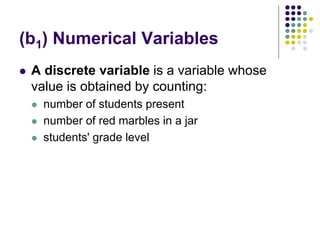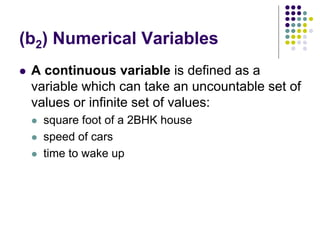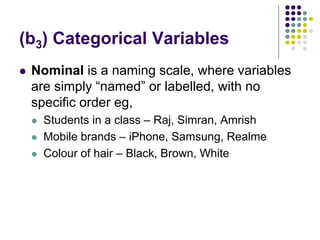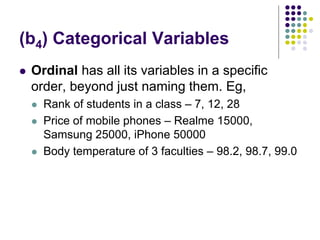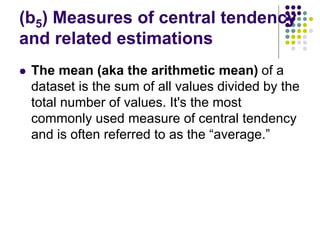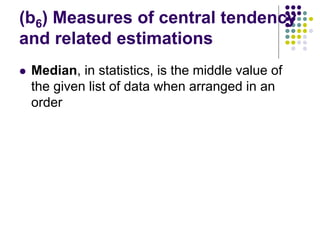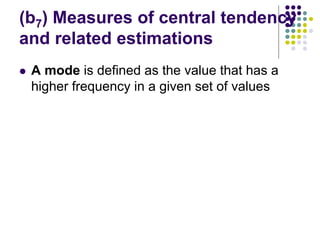1 de 11

### (b) Data Types and Measures of Central Tendency.ppt

1. Marketing Analytics (b) Data Types and Measures of Central Tendency Dr. Pranab S Deb Faculty – Sri Balaji University, Pune
2. (b1) Numerical Variables  A discrete variable is a variable whose value is obtained by counting:  number of students present  number of red marbles in a jar  students' grade level
3. (b2) Numerical Variables  A continuous variable is defined as a variable which can take an uncountable set of values or infinite set of values:  square foot of a 2BHK house  speed of cars  time to wake up
4. (b3) Categorical Variables  Nominal is a naming scale, where variables are simply “named” or labelled, with no specific order eg,  Students in a class – Raj, Simran, Amrish  Mobile brands – iPhone, Samsung, Realme  Colour of hair – Black, Brown, White
5. (b4) Categorical Variables  Ordinal has all its variables in a specific order, beyond just naming them. Eg,  Rank of students in a class – 7, 12, 28  Price of mobile phones – Realme 15000, Samsung 25000, iPhone 50000  Body temperature of 3 faculties – 98.2, 98.7, 99.0
6. (b5) Measures of central tendency and related estimations  The mean (aka the arithmetic mean) of a dataset is the sum of all values divided by the total number of values. It's the most commonly used measure of central tendency and is often referred to as the “average.”
7. (b6) Measures of central tendency and related estimations  Median, in statistics, is the middle value of the given list of data when arranged in an order
8. (b7) Measures of central tendency and related estimations  A mode is defined as the value that has a higher frequency in a given set of values
9. (b8) Measures of central tendency and related estimations  Variance is a measure of dispersion that takes into account the spread of all data points in a data set  In statistics, variance measures variability from the average or mean, where: s2 - sample variance xi – value of 1 observation x- - mean value of all observations n – number of observations
10. Q & A
11. Thanks